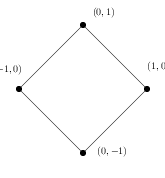UNB/ CS/ David Bremner/ teaching/ cs6375/ sample-questions

## Midterm 1

1. You run factory that makes 3 kinds of chocolate, milk, semi-sweet, and dark. Each kind of chocolate sells for a different price per kilogram and uses a different amount of milk, sugar, and cocoa mass. Formulate an LP to choose the optimal product mix to manufacture for give raw material prices.

2. For each of the following modifications to a hypothetical LP, explain the possible changes to solution of the LP.

• Adding a constraint
• Removing a constraint
• Negating the coefficients of the objective function
3. For the following LP, prove that in an optimal solution `eᵢ=|xᵢ|`

``````  min ∑ eᵢ
eᵢ ≥ -xᵢ
eᵢ ≥  xᵢ
Ax ≤ b
``````
4. The L₁ or taxicab distance between points `p=(p₁,p₂)` and `q=(q₁,q₂)` is defined as

d₁(p,q) = |p₁-q₁|+|p₂-q₂|

• Give a set of linear inequalities to enforce the constraint that d_1(p,(0,0))≤ 1. The feasible region is drawn below. Note that a simple solution without extra variables exists.5. Given a set of 2D points, write a linear program to compute the size of the smallest axis aligned square (not necessarily centred at the origin) that encloses the points.

6. You run a business which buys 16' lengths of lumber from a wholesaler. You receive orders from customers for 4', 8', and 12' lengths of lumber. Explain how to write an integer program to minimize the number of 16' lengths needed fulfil a given set of orders.

7. Consider the following integer program for finding the maximum independent set in a graph `G = (V,E)`.

``````  max ∑ xᵥ
xᵤ + xᵥ ≤ 1     ∀ (u,v) ∈ E
xᵥ ∈ {0,1}
``````

Prove that the LP relaxation for this integer program always has a solution with objective value at least |V|/2. Given an example of a graph where this corresponds to the optimal value of the integer program.

8. Consider two LPs with the same objective function

``````max cᵀx
Ax≤ a

max cᵀx
Bx≤ b
``````

Let `xᴬ` and `xᴮ` be the corresponding optimal solutions. Let `z` be the optimal be the optimal solution to the LP

``````max cᵀx
Ax≤ a
Bx≤ b
``````

For each of following inequalities, explain if it sometimes holds, always holds, or never holds.

``````cᵀxᴬ < cᵀz

min(cᵀxᴬ, cᵀxᴮ) > cᵀz
``````
9. Consider the following LP, related to a graph `G=(V,E)`

``````max ∑ xₑ
∑{xₑ | u ∈ e}≤ 1     ∀ u ∈ V
0 ≤ xₑ ≤ 1
``````

Prove that for an optimal solution `x*` the edge set `E*` of non-integral edges does not contain an odd maximal path.

## For test 2

1. Γ be the LP `max c^Tx s.t. Ax = b, x ≥ 0`. Let `x'` be a feasible solution to this LP. Let `K = { j | x'ⱼ > 0 }`.

• Suppose `A_K` does not have full column rank. Show that there exists `y≠0` such that `Ay=0`
• Suppose the vector `y` above satisfies `y ≥ 0`, `cᵀy > 0`. Prove that `Γ` is unbounded.
2. If `B` is a feasible basis for system `Ax = b, x >= 0`, derive the formula for the corresponding basic feasible solution.

3. Let Γ be the LP `max c^Tx s.t. Ax = b, x ≥ 0`. Let B define a feasible basis of Γ . Define

``````c̃_j = 0   if j ∈ B
= -1  otherwise
``````

Let `x'` the basic feasible solution corresponding to B. Prove that `c̃ᵀx' ≥ c̃ᵀy` for all feasible `y`.

4. Prove that (feasible) simplex tableaus are in one to one correspondence with feasible bases.

5. Let `Γ` be the LP `max cᵀx s.t. Ax ≤ b`.

• prove that set of all optimal solutions to `Γ` is a convex set

• Let `x'` be a basic feasible solution of `Γ`. Let `J` denote the set of `n` linearly independent rows `aⱼ x ≤ b` satisfied with equality. Suppose `c = ∑ {aⱼ | j∈J}`. Show that for all feasible `y ≠ x'`, `cᵀy < cᵀx'` .

## For final

1. Consider the LP `max cᵀx Ax = b, x >= 0`. Prove that if there is some `w` such that `Aw=0` and `cᵀw > 0`, and the LP is feasible, then the LP is unbounded.

2. Give an example of a simplex tableau that is both degenerate and optimal. Explain your answer.

3. Show how to use the simplex method to test if a system of linear inequalities is feasible.

4. Show that for any non-negative `x` and `y` such that `Ax ≤ b` and `Aᵀy ≥ c`, it also holds that `cᵀx ≤ bᵀy`

5. Show how to reduce solving an LP to testing a system of inequalities for feasibility.

6. Consider the following LP.

``````max x₁ + x₂ + x₃ s.t.
x₂ + 0.5x₃ ≤ 1
−2x₁ + x₃ ≤ 0
x₁ + 0.5x₃ ≤ 1
−2x₂ + x₃ ≤ 0
x₁ , x₂ , x₃ ≥ 0
``````
• Prove that x = (1/2, 1/2, 1) and y = (1, 0, 1, 0) are primal–dual optimal solutions for this LP.

• Prove that, under the assumption that `xᵢ` are integer, `x₃≤0` is a valid Chvátal-Gomory cutting plane for this LP. (this requires guessing a good set of row multipliers)

7. Consider the following integer program.

``````   max x₂
−x₁  + 2x₂ ≤ −1/2
x₁ + 2x₂ ≤ 5/2
x₁ , x₂ ≥ 0
xᵢ integer
``````
• The LP relaxation has optimal tableau

``````  x₁ = 3/2 - 1/2 x₃ - 1/2 x₄
x₂ = 1/2 - 1/4 x₃ - 1/4 x₄
z  = 1/2 - 1/4 x₃ - 1/4 x₄
``````

Derive cutting planes from this tableau. Explain your answer.

• Draw the branch and bound tree, indicating what constraints are introduced during branching. Hint: draw a picture of feasible region of the initial relaxation.